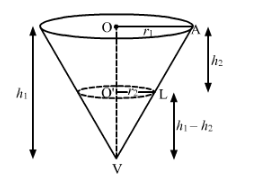# The height and radius of the cone of which the frustum`
Question:

The height and radius of the cone of which the frustum is a part are h1 and r1 respectively. If h2 and r2 are the heights and radius of the smaller base of the frustum respectively and h2 : h1 = 1 : 2, then r2 : r1 is equal to

(a) 1 : 3

(b) 1 : 2

(c) 2 : 1

(d) 3 : 1

Solution:Since,

$\triangle A O V$ and $L O^{\prime} V$ are similar triangles,

i.e., In $\triangle A O V$ and $L O^{\prime} V$

$\frac{O A}{O^{\top} L}=\frac{O V}{O^{\prime} V}$

$\Rightarrow \frac{r_{1}}{r_{2}}=\frac{h_{1}}{h_{1}-h_{2}}$

$\Rightarrow\left(h_{1}-h_{2}\right) r_{1}=h_{1} r_{2}$

$\Rightarrow r_{1} h_{1}-r_{1} h_{2}=h_{1} r_{2}$

$\Rightarrow r_{1} h_{1}-h_{1} r_{2}=r_{1} h_{2}$

$\Rightarrow h_{1}\left(r_{1}-r_{2}\right)=r_{1} h_{2}$

$\Rightarrow \frac{\left(r_{1}-r_{2}\right)}{r_{1}}=\frac{h_{2}}{h_{1}}$

$\Rightarrow \frac{\left(r_{1}-r_{2}\right)}{r_{1}}=\frac{1}{2}$

$\Rightarrow 1-\frac{r_{2}}{r_{1}}=\frac{1}{2}$

$\Rightarrow \frac{r_{2}}{r_{1}}=1-\frac{1}{2}=\frac{1}{2}$

Thus, $r_{2}: r_{1}=1: 2$

Hence, the correct answer is choice (b).